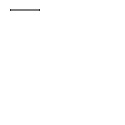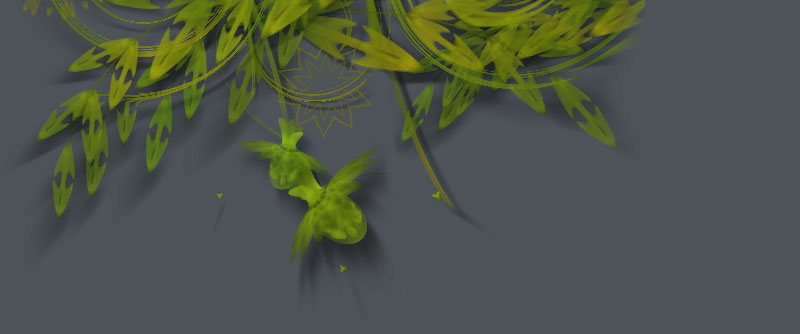### Reference | drawpath()

Syntax
`drawpath(path)`

DescriptionDraws a path to the screen. A path is a series of lines and curves defined between beginpath() and endpath(). Normally, endpath() draws the path to the screen, unless when calling endpath(draw=False). The path can then be assigned to a variable, and this variable used as a parameter for drawpath().

Note: if you have one path that you want to draw multiple times with drawpath(), for example each with its own rotation and position, you need to supply a copy:
drawpath(path.copy())

Tutorial Paths

Example```stroke(0.2) beginpath(10, 10) lineto(40, 10) p = endpath(draw=False) drawpath(p)```